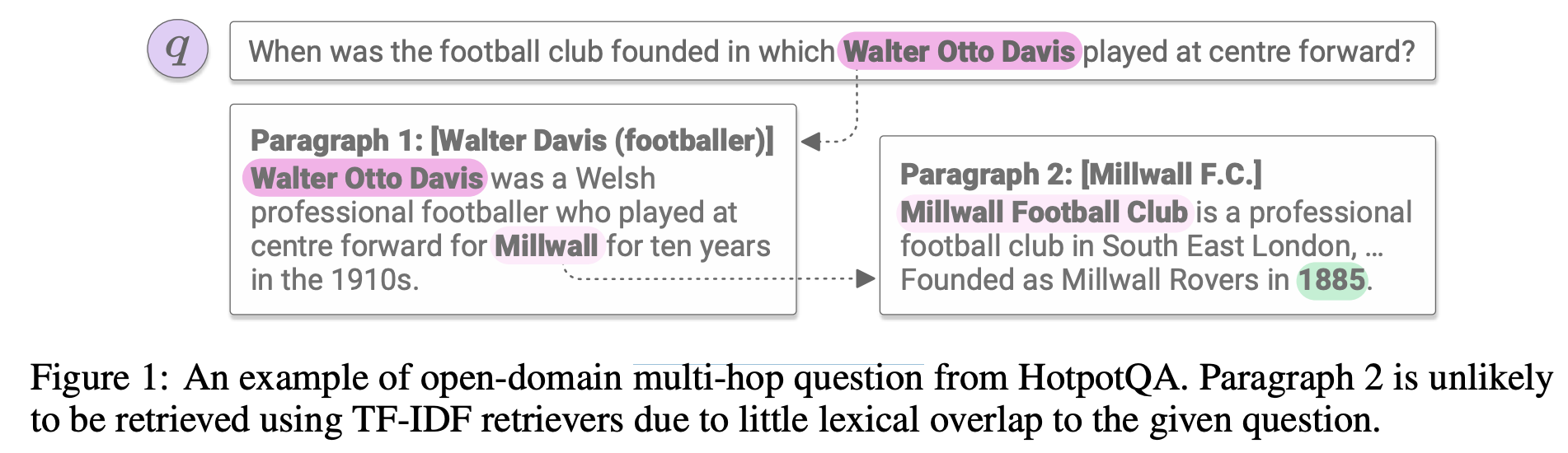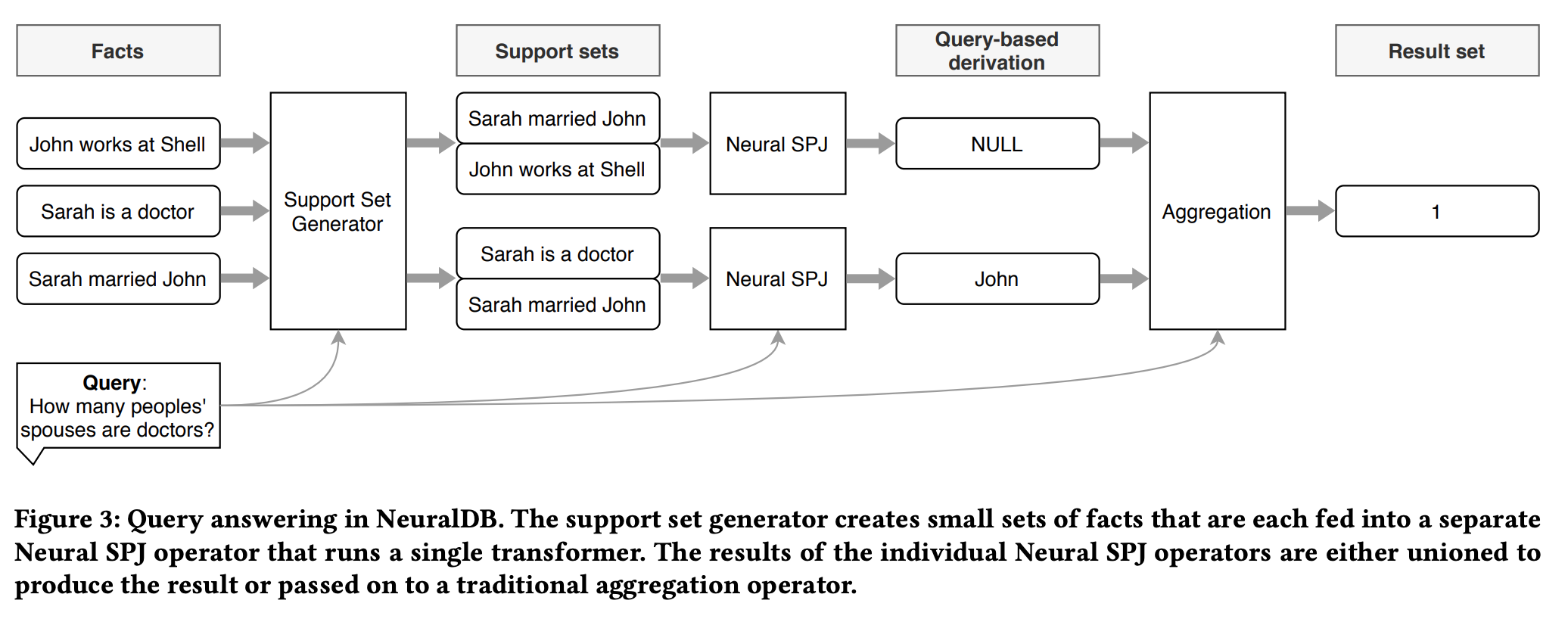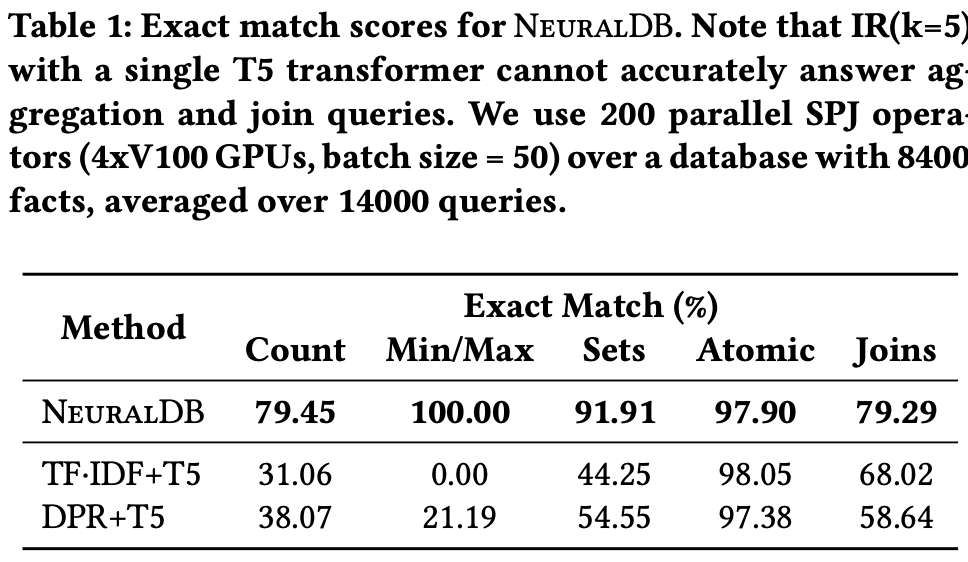# From Natural Language Processing to Neural Databases

27 Oct 2021

From Natural Language Processing to Neural Databases, James Thorne et al., VLDB 2021

I came across a very interesting paper when I was browsing the VLDB proceedings this year. Question Answering (QA) has been a hot topic in Natural Language Processing (NLP) research, but if we see this problem from a Database research perspective, we usually care about the scale of the problem. This work seems to push the scale of the QA problem to a new limit. Given a query in a short sentence format, can we obtain answers that come from (1) thousands of facts from a database and (2) involve a series of select-project-join-aggregate operations? This might seem like a small querying problem at first glance. However, we need to understand some limitations from the NLP side. First, the language models like Transformer usually take a small input. In the query answering scenario, the models are trained with $$<D,Q,A>$$ triplets, where $$D$$ is a set of facts, $$Q$$ is the query sentence, and $$A$$ is the answer. If the whole database’s facts are fed into the Transformer model, the size of $$D$$ will be super large, and the model will sort of “explode” since the memory requirement is quadratic to the size of the input. Second, the Transformer models work well in identifying the sentences that provide the answer but they usually do not address the scenario where there is a need to synthesize multiple sentences to obtain the answer. Thus, the case where the facts need to be joined/ aggregated will not function well for the language models.

The work addresses the query answering problem by breaking them into three separate tasks. First, it trains a model called Support Set Generator (SSG), which provides a multi-hop answering capability to output subsets of facts that are related to the question. More in-depth details of how it works can be found in this ICLR paper. Below is an example of multi-hop answering:Once the whole fact table is reduced into small subsets of facts $$D_1, D_2,...$$, together with the query $$Q$$ we can feed multiple inputs $$(D_1,Q), (D_2,Q), ...$$ (in parallel) to a trained or pre-trained Transformer to get a set of high-quality answers. To aggregate the answers with aggregate operators like Min/Max, we need to train a classifier that maps a query to an aggregation function. The whole pipeline is shown below:The experiment shows that such a pipeline performs well when compared against a single Transformer with some simple single-hop answering setups, which is shown in the table below:It is not clear to me that whether the approach works well in different scenarios since all three components in the pipeline are black-box models, and the experiment is only done with generated sentences from a small fact table. However, it explains clearly that how to integrate language models in a complicated relational database setting, which inspires lots of possible future directions.